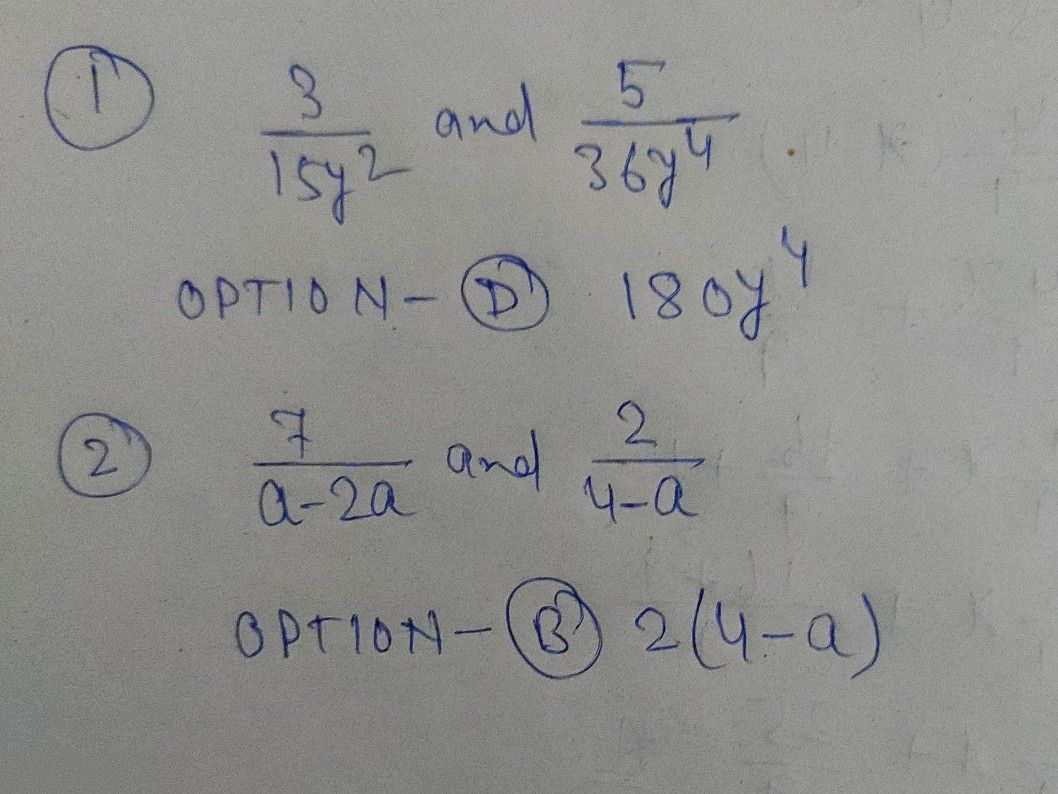Symbol
Problem$1$ Give the Least Common Denominator $\left(tcD\right)$ of $\dfrac {3} {15y^{2}}$ and $\dfrac {5} {36y^{4}}$ A. $36y$ $C$ $90y^{2}$ B. $36y^{4}$ $0$ $180y^{4}$ $2$ Find the LCD of $\dfrac {7} {0-2a}$ and $\dfrac {2} {4-a}$ A. $\left(4-a\right)$ $C1$ $\left(a^{2}+64\right)$ B. $2\left(4-a\right)$ D. $\left(64-a^{2}\right)$
7th-9th grade
Algebra
Search count: 113
SolutionQanda teacher - Ranjeet